telescopeѲptics.net          ▪▪▪▪                                             CONTENTS

# 10.2.3.1. Maksutov corrector surface radii

Due to enormous amount of spherical aberration generated by strongly curved surfaces of achromatic meniscus corrector in the Maksutov camera, it is very sensitive to both, surface radii differential and variation in corrector thickness. Also, significant higher-order spherical aberration it generates makes lower-order expression alone insufficient for determining corrector properties needed to minimize spherical aberration of the mirror. Thus, there is no simple way to find out specific corrector radii and thickness for a given mirror. However, it is possible to arrive at a good approximation, either for informative purposes, or to be used as a starting point in designing corrector.

Expressing meniscus center thickness t and front surface radius of curvature R1 in terms of the mirror radius of curvature R as t=-τR (which determines parameter τ as numerically positive), and R1=κR allows for establishing relation between these two parameters based on Eq. 125, as [κ-(1-1/n2)τ]κ3=-(n+2)(n-1)2τ/2n3. With τ being much smaller than κ, neglecting [1-(1/n2)]τ leads to the approximation κ4~(n+2)(n-1)2τ/2n3 and the first iteration for needed value of the first radius for corrected lower-order spherical aberration:where n is, as before, the corrector glass refractive index, and τ its relative center thickness t/R.

Once the initial approximation of R1 is known, a correction factor compensating for the neglected value can be applied, giving closer approximation of the needed first radius as: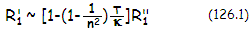The original corrector thickness, chosen by Maksutov, was t=D/10. Thicker corrector reduces somewhat residual spherical aberration, but at a price of increased lateral color. Optimum thickness is, as noted by Rutten and Venrooij (p101), probably some 50% greater, thus t~D/7 (either can be easily expressed in terms of mirror radius of curvature R for any specific mirror).

As already mentioned, meniscus corrector with these parameters nearly corrects for third-order spherical aberration of a mirror of the radius of curvature R. However, due to strongly curved surfaces, there is always residual fifth-order aberration present. It is negligible compared to the lower-order aberration of the mirror, but once it is near corrected, the higher-order aberration becomes a factor. To minimize the aberration, the two need to be optimally balanced, by combining their near-equal amounts of opposite signs. Due to this, as well as due to the approximations made for simplicity, near-optimum first radius R1 will be nominally somewhat smaller than what is indicated by Eq. 126-126.1.

Typically, best radius of curvature for the front meniscus surface is closely approximated by R1~0.94R'1. Nearly as good final approximation is given by R1~0.9R''1, which indicates that the first iteration is a sufficiently good basis for estimating the needed first radius.

Once R1 is known, the second radius is obtained from: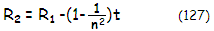which in turn determines the thickness t in terms of the radii and index of refraction, as given with Eq. 124.

Keep in mind that the corrector is convex toward the mirror, thus with the radii nominally negative. Since the thickness is positive, this means that R2 is weaker than R1, and the meniscus is a weak negative lens, thicker at the edge than at the center.

With these parameters, a single-mirror Maksutov system is roughly corrected; however, the approximate character of the procedure, combined with the high system sensitivity to changes in optimum meniscus parameters, require verification and likely correction. Further detailed optimization improves quality level, but the main benefit is achieved by balancing the two forms of spherical aberration. Final optimization is best done with the help of ray tracing software, as illustrated on FIG. 183.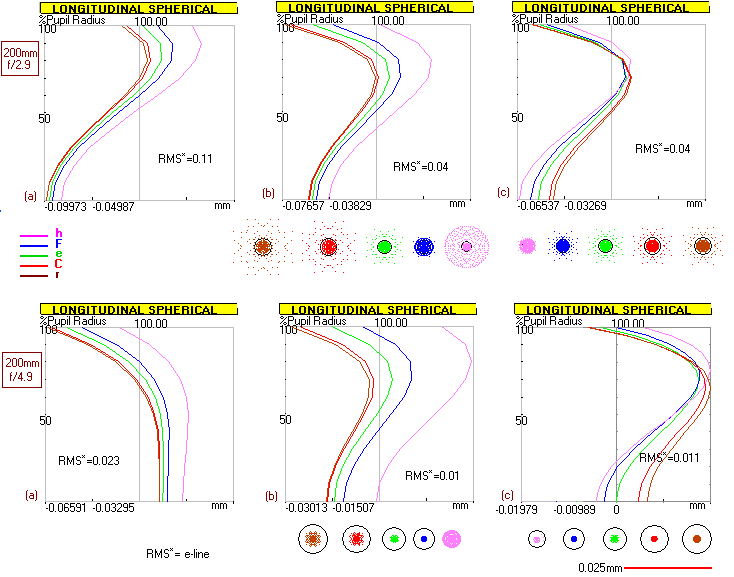FIGURE 183: TOP: Illustration of the process of finding best meniscus parameters. For D=200mm f/3 sphere with t=25mm and BK7 glass, 0.9R''1=-249mm which, taken as R1, with R2 obtained from Eq. 128, gives an f/2.9 system ready for optimization (a). Strengthening the radii to R1/R2=-246.25/-260.4 results in near-optimum correction for the central line (b). However, while the paraxial foci nearly coincide, the outer zonal foci are more widely separated, resulting in less than optimum sphero-chromatic correction. Optimizing is accomplished by changing the radii relation to R2=R1-[1-(1/n2)]t/0.97. This results in the outer zonal foci coming close together (c). BOTTOM: The other example is for a D=200mm f/5 sphere with t=20mm, which is actually a telescope in the Newtonian configuration. First iteration (0.9R"1) already results in a very useable system (a). However, it can and should be optimized through the same procedure. Bringing all colors as close as possible would require slightly stronger radii (-352.5/-364 vs. -357.5/-369.2mm) but the gain wouldn't be detectable in field use).         SPEC'S (optimized only)

It should be noted that the above approximation is for the corrector-to-mirror separation of ~2R/3, needed for zero system coma. With the change in the position of the corrector, its radii needed for zero system spherical aberration also change. This is due to Maksutov corrector having relatively significant power (much more so than the Schmidt corrector), which effectively changes for the mirror with their separation. In general, decrease in separation increases the effective corrector power, requiring weaker radii, and vice versa. Also, with large mirror focal ratios (~f/3 and larger), higher-order coma of the corrector increases sufficiently to require somewhat smaller zero-coma corrector-to-mirror separation.

In practice, there is no need to calculate for best paraxial correction, knowing that the optimum overall correction is different. The correction factor for the optimum overall system correction can be applied directly to R"1 given by Eq. 126, in an empirical relation taking into account slight variations due to the changes in relative thickness τ=t/R, R being the mirror radius of curvature, and mirror focal ratio F. This gives the meniscus radii approximation as: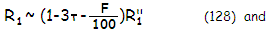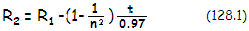with n being the glass refractive index, F the mirror focal ratio and t, as before, the nominal meniscus center thickness.

This first radii approximation should bring the wavelengths quite close together, with relatively small residual spherical aberration present. If the blue is farther away than the red, while spherical aberration is relatively low, both radii need to be strengthened, while keeping the differential nearly unchanged. Opposite if the red focuses farther away. When the wavelengths are close together, spherical aberration is minimized by small adjustment in one of the corrector radii (if the residual spherical is significant, then it needs to be minimized first, by finding needed radius differential; after that the radii are changed as needed, with relatively small changes in their differential, if needed, until both chromatic and spherical aberration are minimized).

Evidently, needed first radius of the corrector becomes slightly stronger as the relative corrector thickness and mirror F-number increase. The 0.97 factor is not strictly fixed; it determines the level of best chromatic correction, not necessarily the very point of numerical maximum. Slight deviations may offer correction modes more appropriate in some instances, but the difference - or benefit, if any - is typically very small.